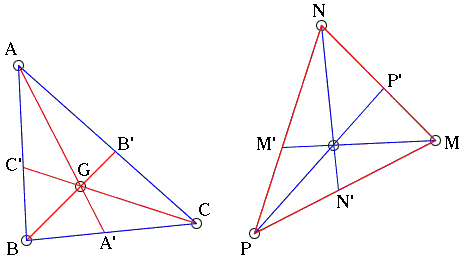# Maxwell Theorem via the Center of Gravity

### Michel Cabart 30 April, 2008

Below we offer a proof of Maxwell's theorem that is based on the notion of barycenter. Maxwell's theorem states the following fact:

Given ΔABC and a point G, the sides of ΔMNP are parallel to the cevians in ΔABC through G. Then the cevians in ΔMNP parallel to the sides of ΔABC are concurrent.Below, the vector joining point A to B will be written in bold, so that, for example, AB = - BA.

In ΔABC with A', B', C' on sides opposite the vertices A, B, and C, the fact that the cevians AA', BB', CC' concur in point G is equivalent to either of the two conditions:

 There is a triple of real numbers (a, b, c), unique up to a non-zero factor, such that (1) aGA + bGB + cGC = 0 There is a triple of real numbers (a, b, c), unique up to a non-zero factor, such that (2) A' = Z(B, b; C, c) B' = Z(A, a; C, c) C' = Z(A, a; B, b),

where Z(X, x; Y, y) denotes the barycenter of two material points X and Y with masses x at X and y at Y.

Let's suppose lines AA', BB' and CC' intersect.

Step 1: NP, PM, MN are parallel to GA, GB, GC means there exists x, y, z such that NP = xGA, PM = yGB, MN = zGC. As NP + PM + MN = 0, xGA + yGB + zGC = 0. A comparison with (1) shows that (x, y, z) is a multiple of (a, b, c). We can assume (x, y, z) = (a, b, c). Thus NP = aGA, PM = bGB, MN = cGC.

Step 2: MM', NN', PP' are parallel to BC, AC, AB meaning there is (m, n, p) such that MM' = mBC, NN' = nAC, PP' = pAB. The first equality yields

MM' = m(GC - GB) = (m/c)MN + (m/b)MP

so that

M' = Z(N, 1/c; P, 1/b) = Z(N, b; C, c)

by multiplying by bc. Similarly,

N' = Z(M, a; P, c) and
P' = Z(M, a; N, b).

This proves the theorem thanks to (2).### Barycenter and Barycentric Coordinates